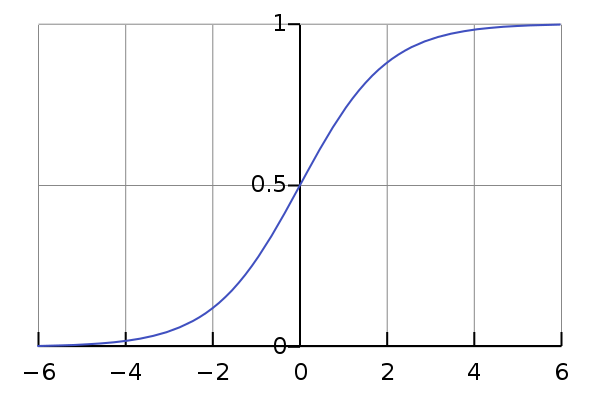# Is ReLU After Sigmoid Bad?

Recently [we] were analyzing how different activation functions interact among themselves, and we found that using relu after sigmoid in the last two layers worsens the performance of the model.By Nishant Nikhil, IIT Kharagpur

There was a recent blog post on mental models for deep learning drawing parallels from optics [link]. We all have intuitions for few models but it is hard to put it in words, I believe it is necessary to work collectively for this mental model.Sigmoid graph from wikipedia

Recently I and Rajasekhar (for a KWoC project) were analyzing how different activation functions interact among themselves, and we found that using relu after sigmoid in the last two layers worsens the performance of the model. We use the MNIST dataset and a four-layered fully connected network, the first layer is the input layer of 784 dimensions, then the second layer is a hidden layer of 500 dimensions, after which another hidden layer of having 256 dimensions and finally an output layer of 10 dimensions. Except for the input layer we use a non-linearity on each layer’s output. As we restrict our study to four activation functions(ReLU, Sigmoid, Tanh, SeLU), we can construct 64 different models by the different combinations of the activation functions. We use stochastic gradient descent in all of the models with a learning rate of 0.01 and momentum of 0.5. We use cross-entropy loss and a batch size of 32 in all our experiments. We ran experiments for each of the models 9 times and the mean and standard deviation of accuracy are shown in the table at [nishnik/sherlocked]. I would give a brief summary here:

1. If the first layer has relu activation, second and third layer have any combination of (relu, tanh, sigmoid, relu) except for (sigmoid, relu) then the mean test accuracy is more than 85%. For (relu, sigmoid, relu) we get an average test accuracy of 34.91%
2. If the first layer has tanh activation, second and third layer have any combination of (relu, tanh, sigmoid, relu) except for (sigmoid, relu) then the mean test accuracy is more than 86%. For (tanh, sigmoid, relu) we get an average test accuracy of 51.57%
3. If the first layer has sigmoid activation, second and third layer have any combination of (relu, tanh, sigmoid, relu) except for (sigmoid, relu) then the mean test accuracy is more than 76%. For (sigmoid, sigmoid, relu) we get an average test accuracy of 16.03%
4. If the first layer has selu activation, second and third layer have any combination of (relu, tanh, sigmoid, relu) except for (sigmoid, relu) then the mean test accuracy is more than 91%. For (selu, sigmoid, relu) we get an average test accuracy of 75.16%
5. Also the variance in the accuracy is high if the last two layers have (sigmoid, relu)

We have conducted experiments on CIFAR-10 also and the results are like-wise [link] (Sorry for the bad formatting). In every case when the last two activations are (sigmoid, relu) the accuracy is 10% otherwise the accuracy ≥ 50%.

Then we conducted experiments with using batch-norm in each layer. And the accuracy increased substantially, same as the other combinations. [Results on MNIST]. Also just using batchnorm on the last layer works like charm to make the model learn.

So for (sigmoid, relu) in the last two layers, the model is not able to learn, i.e. the gradients are not back propagated well. Either (sigmoid(output_2)*weigth_3 + bias_3) < 0 for most cases or sigmoid(output_2) is reaching the extremes (vanishing gradient). I am still doing experiments on these two. Suggest me something at twitter.com/nishantiam or create an issue on [nishnik/sherlocked].

Bio: Nishant Nikhil is an undergraduate student at IIT Kharagpur interested in Deep Learning. You can follow him on Twitter (@nishantiam) or check out his GitHub at github.com/nishnik.

Original. Reposted with permission.

Related:

• Neural Network Foundations, Explained: Activation Function
• An Intuitive Guide to Deep Network Architectures
• Neural Network Foundations, Explained: Updating Weights with Gradient Descent & Backpropagation